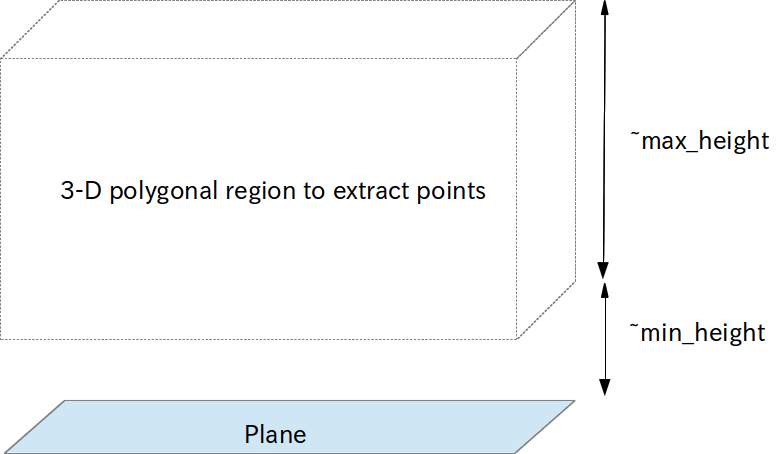# MultiPlaneExtraction¶Extract the points above the planes between ~min_height and ~max_height.

## Subscribing Topics¶

• ~input (sensor_msgs/PointCloud2):

Input pointcloud.

• ~indices (jsk_recognition_msgs/ClusterPointIndices)

• ~input_polygons (jsk_recognition_msgs/PolygonArray)

• ~input_coefficients (jsk_recognition_msgs/ModelCoefficientsArray):

The input planes.

If ~use_indices parameter is false, ~indices is used.

If ~use_coefficients parameter is false, ~coefficients is not used. (Instead ~use_sensor_frame should be enabled in order to determine normal direction of planes.)

## Publishing Topics¶

• ~output (sensor_msgs/PointCloud2):

Pointcloud above the planes between ~min_height and ~max_height.

• ~output_nonplane_cloud (sensor_msgs/PointCloud2):

Pointcloud above the planes is not between ~min_height and ~max_height.

• ~output/indices (pcl_msgs/PointIndices)

PointIndices of points which are between ~min_height and ~max_height.

## Parameters¶

• ~keep_organized (Bool, default: True)

Keep organized point cloud or not.

• ~min_height (Double, default: 0.0)

• ~max_height(Double, default: 0.5)

Minimum and maximum height of 3-D polygonal region to extract points.

• ~max_queue_size (Integer, default: 100)

Queue length for subscribing topics.

• ~use_indices (Bool, default: True)

Use indices of planar regions to filter if it’s set true. You can disable this parameter to filter pointcloud which is not the same pointcloud to segment planes

• ~magnify (Double, default: 0.0)

Magnify planes by this parameter. The unit is m.

• ~use_async (Boolean, default: False)

Approximate sync input topics.

• ~use_coefficients (Bool, default: False)

Use coefficients topic to determine viewpoint. For avoiding plane flipping, either this parameter or ~use_sensor_frame should be enabled.

• ~use_sensor_frame (Boolean, default: False)

Set viewpoint as a TF sensor frame. If this parameter is enabled, ~use_coefficients is ignored and handled as false.

• ~sensor_frame (String, default: head_root)

Specify frame_id of sensor origin. This parameter is enabled only when ~use_sensor_frame is enabled.

## Issues¶

• Normal direction of planes

Normal directions of each planes are computed as followings:

use_sensor_frame: false use_sensor_frame: true
use_coefficients: false PCL computes z-axis internally (it occurs flipping. warning shows up) Determine z-axis direction to TF frame
use_coefficients: true Determine z-axis from coefficients topic N/A (This condition is invalid. ~use_coefficients is disabled in this case. Also warning shows up)

Both ~use_sensor_frame and ~use_coefficients is disabled by default, which means the normal directions are computed individually on every callback and can be flipped on the continuous time domain.

Generally it’s better to set either ~use_sensor_frame or ~use_coefficients as true for stable detection.

## Sample¶

roslaunch jsk_pcl_ros sample_multi_plane_extraction.launch